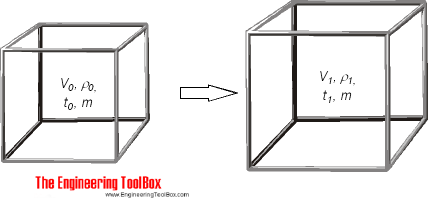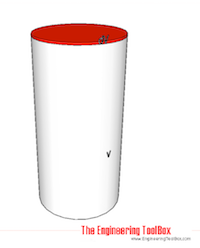Engineering ToolBox - Resources, Tools and Basic Information for Engineering and Design of Technical Applications!

# Volumetric (Cubic) Thermal Expansion

## Volumetric temperature expansion calculator.Specific volume of a unit can be expressed as

v = 1 / ρ = V / m                                                          (1)

where

v = specific volume (m3/kg, ft3/lb)

ρ = density (kg/m3, lb/ft3)

V = volume of unit (m3, ft3)

m = mass of unit (kg, lb)

The change in the units volume when temperature changes can be expressed as

dV = V0 β (t1 - t0)                                                        (2)

where

dV = V1 - V0 = change in volume (m3, ft3)

β = volumetric temperature expansion coefficient (m3/m3 oC, ft3/ft3 oF)

t1 = final temperature (oC, oF)

t0 = initial temperature (oC, oF)

The density of a fluid when temperature changes can be expressed as

ρ1 = m / V0 (1 + β (t1 - t0))

= ρ0 / (1 + β (t1 - t0))                                                (3)

where

ρ1 = final density (kg/m3, lb/ft3)

ρ0 = initial density (kg/m3, lb/ft3)

### Online Thermal Cubic Expansion Calculator - Expansion coefficient and Temperatures

Be aware that the expansion coefficient for some liquids - like water - may vary with temperature. The calculator below is generic and can be used for metric and imperial units as long as the use of units is consistent.

V0 - initial volume (m3, ft3, gallons ..)

β - volumetric temperature expansion coefficient (1/oC, 1/oF)

t0 - initial temperature (oC, oF)

t1 - final temperature (oC, oF)

Note that the volumetric expansion coefficient used in the calculator is constant. If you want to calculate volumetric change for a liquid over a temperature range where the volumetric expansion coefficient for the liquid changes a lot - interpolate the coefficient values, or split the calculation in the different temperature ranges. Example - water is a liquid where the volumetric expansion coefficient changes a lot with temperature. Water has its highest density and smallest volume at 4oC (39.2 oF). The volumetric coefficient for water is negative below 4oC and indicates that the a volume decreases when temperature moves from 0oC (32 oF) to 4oC.

### Online Thermal Cubic Expansion Calculator - Densities

This calculator can be used to calculate expansion volume when initial volume and initial and final densities for the liquid are known

V0 - initial volume (m3, ft3)

ρ0 - initial density (kg/m3, lb/ft3)

ρ1 - final density (kg/m3, lb/ft3)

### Volumetric Temperature Coefficients - β - for some Fluids

• water at 0oC: -0.000050 (1/oC)
• water at 4oC: 0 (1/oC)
• water at 10oC: 0.000088 (1/oC)
• water at 20oC: 0.000207 (1/oC)
• water at 30oC: 0.000303 (1/oC)
• water at 40oC: 0.000385 (1/oC)
• water at 50oC: 0.000457 (1/oC)
• water at 60oC: 0.000522 (1/oC)
• water at 70oC: 0.000582 (1/oC)
• water at 80oC: 0.000640 (1/oC)
• water at 90oC: 0.000695 (1/oC)
• ethyl alcohol: 0.00109 (1/ oC), 0.00061 (1/oF)
• oil: 0.00070 (1/oC), 0.00039 (1/oF)

### Convert between Metric and Imperial Volumentric Temperature Coefficients

• 1 (1/oC) =  0.56 (1/oF)
• 1 (1/oF) =  1.8 (1/oC)

### Example - Cubic Expansion of Oil100 liters - 0.1 m3 - of oil with volumetric expansion coefficient 0.00070 1/oC is heated from 20oC to 40oC. The volumetric expansion can be calculated using equation (2)

dV = (0.1 m3) (0.00070 1/oC) ((40 oC) - (20 oC))

= 0.0014 m3

= 1.4 liter

The final volume is

100 liters + 1.4 liters = 101.4 liters

### Example - Cubic Expansion of Oil

30 US gallons of oil is heated from 70oF to 100oF. The volumetric expansion can be calculated using equation (2)

dV = (30 gallons) (0.00039 1/oF) ((100 oF) - (70 oF))

= 0.351 gallons

The final volume is

30 gallons + 0.351 gallon = 30.351 gallons

## Related Topics

• ### Densities

Densities of solids, liquids and gases. Definitions and convertion calculators.
• ### Heating

Heating systems - capacity and design of boilers, pipelines, heat exchangers, expansion systems and more.
• ### Thermodynamics

Work, heat and energy systems.
• ### Water Systems

Hot and cold water service systems - design properties, capacities, sizing and more.

## Related Documents

• ### Air - Thermophysical Properties

Thermal properties of air at different temperatures - density, viscosity, critical temperature and pressure, triple point, enthalpi and entropi, thermal conductivity and diffusivity and more.
• ### Circular Ring - Temperature Expansion

Calculate ring (or pipe) diameter expansion or contraction when temperature changes.
• ### Ethylene Glycol Heat-Transfer Fluid Properties

Properties like freezing point, viscosity, specific gravity and specific heat of ethylene glycol based heat-transfer fluids, or brines.
• ### Gravity Heating System

The density difference between hot and cold water is the circulating force in a self circulating gravity heating system.
• ### Hot Water Expansion Tanks - Sizing

Required hot water expansion volume in open, closed and diaphragm tanks.
• ### Linear Thermal Expansion

Online linear temperature expansion calculator.
• ### Liquids - Densities vs. Pressure and Temperature Change

Densities and specific volume of liquids vs. pressure and temperature change.
• ### Liquids - Volumetric Expansion Coefficients

Volumetric - or cubical - expansion coefficients for common liquids.
• ### Propylene Glycol based Heat-Transfer Fluids

Freezing points of propylene glycol based heat-transfer fluids suitable for the food processing industry.
• ### Shrink-Fits Assembly

Assembly temperatures for shrink-fits.
• ### Solids - Volume Temperature Expansion Coefficients

Cubical expansion coefficients for solids.
• ### Thermal Expansion - Linear Expansion Coefficients

Linear temperature expansion coefficients for common materials like aluminum, copper, glass, iron and many more.
• ### Thermal Expansion - Superficial i.e. Area

Superficial i.e area temperature expansion - online calculator.
• ### Water - Thermophysical Properties

Thermal properties of water at different temperatures like density, freezing temperature, boiling temperature, latent heat of melting, latent heat of evaporation, critical temperature and more.

## Engineering ToolBox - SketchUp Extension - Online 3D modeling!

Add standard and customized parametric components - like flange beams, lumbers, piping, stairs and more - to your Sketchup model with the Engineering ToolBox - SketchUp Extension - enabled for use with older versions of the amazing SketchUp Make and the newer "up to date" SketchUp Pro . Add the Engineering ToolBox extension to your SketchUp Make/Pro from the Extension Warehouse !

We don't collect information from our users. More about

## Citation

• The Engineering ToolBox (2009). Volumetric (Cubic) Thermal Expansion. [online] Available at: https://www.engineeringtoolbox.com/volumetric-temperature-expansion-d_315.html [Accessed Day Month Year].

Modify the access date according your visit.

9.19.12